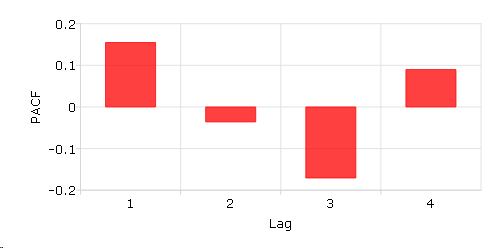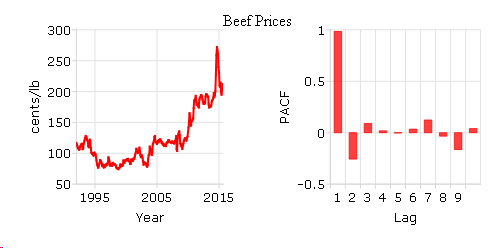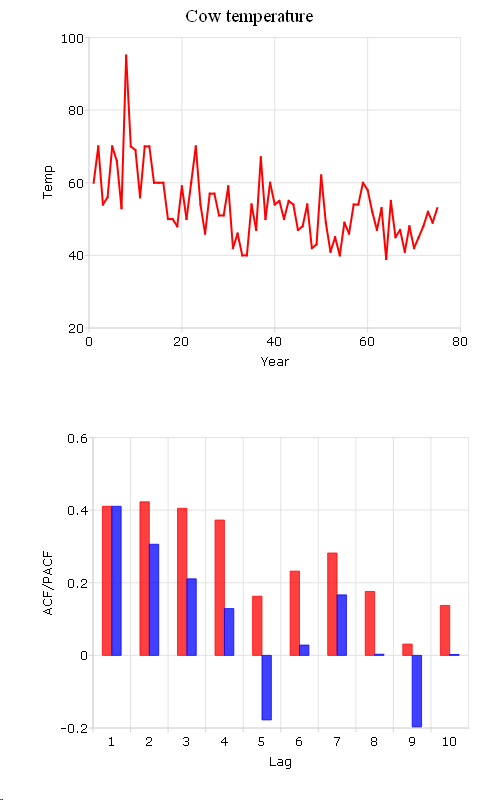# pacf¶

## Purpose¶

Computes sample partial autocorrelations.

## Format¶

rk = pacf(y, k, d)
Parameters
• y (Nx1 vector) – data

• k (scalar) – maximum number of partial autocorrelations to compute. $$0 < k < N$$.

• d (scalar) – order of differencing. If only computing the autocorrelations from the original time series, then d equals 0.

Returns

rk (Kx1 vector) – sample partial autocorrelations.

## Examples¶

### A sample partial autocorrelation function example.¶

// Create short time-series column vector
x = { 12.92,
14.28,
13.31,
13.34,
12.71,
13.08,
11.86,
9.000,
8.190,
7.970,
8.350,
8.200,
8.120,
8.390,
8.660  };

// Maximum number of lags
k = 4;

// Order of differencing
d = 1;

// Calculate and print result of partial autocorrelation function
rk = pacf(x, k, d);

print rk;

  0.15488076
-0.035928234
-0.17063786
0.089875096


Plot the PACF results with plotBar(). Passing in 0 as the first input tells GAUSS to create a sequential series from 1 to the number of elements in rk as the x-tick labels.

plotBar(0, rk);


You can add labels for x-axis and y-axis interactively on the Graphics Page by selecting View > Graph Settings from the main menu. The plot is shown below:### Calculate the partial autocorrelation function (PACF) and plot the results for “beef_prices” data.¶

// Clear out variables in GAUSS workspace
new;

// Create file name with full path
file = getGAUSSHome() $+ "examples/beef_prices.csv"; // Import dataset starting with row 2 and column 2 beef = csvReadM(file, 2, 2); // Max lags k = 10; // Order of differencing d = 0; // Call pacf function beef_pacf = pacf(beef, k, d);  Create a time series plot and sample partial autocorrelation (PACF) plot based on the beef and beef_pacf variables created above: // Time series plot // Declare a plotControl structure struct plotControl ctl; ctl = plotGetDefaults("xy"); // Make a 1 by 2 plot with the time series // plot in the [1,1] location plotLayout(1, 2, 1); // Labels and format settings for 'beef' matrix plot plotSetYLabel(&ctl, "cents/lb"); plotSetXLabel(&ctl, "Year"); plotSetXTicLabel(&ctl, "YYYY"); plotSetXTicInterval(&ctl, 120, 199501); // Time plot with plotTS function plotTS(ctl, 1992, 12, beef); // Making a 1 by 2 plot, the second plot is the PACF plot plotLayout(1, 2, 2); // ACF plot // Fill 'ctl' structure with defaults settings for bar plots ctl = plotGetDefaults("bar"); // Setting labels and format based on 'beef_acf' matrix plotSetYLabel(&ctl, "PACF"); plotSetXLabel(&ctl, "Lag"); plotSetXTicInterval(&ctl, 1, 5); // PACF plot with plotBar function plotBar(ctl, seqa(1, 1, k), beef_pacf);  You can use ‘Add Text’ to type ‘Beef Prices’ as the title in the graphics window. The plot is:### Compare ACF and PACF for “cow” data.¶ new; cls; file = getGAUSSHome()$+ "examples/cows.fmt";

// Import '.fmt' data
load data = ^file;

// Max lags
k = 10;

// Order of differencing
d = 0;

// call pacf function
data_pacf = pacf(data, k, d);

// call acf function
data_acf = acf(data, k, d);


In this example, we compute the ACF and PACF for cow’s temperature and save them in data_acf and data_pacf.

The following code plot autocorrelation (ACF) and sample partial autocorrelation (PACF):

// Compare ACF and PACF for cow's temperature data
// Create sequential numbers
years = seqa(1, 1, rows(data));

// Declare a plotControl structure
struct plotControl cow_ctl;
cow_ctl = plotGetDefaults("xy");

// Set plot title for top graph
plotSetTitle(&cow_ctl, "Cow Temperature");

// Labels and format setting based on 'data_acf' matrix
plotSetYLabel(&cow_ctl, "Temp");
plotSetXLabel(&cow_ctl, "Year");

// Making a 2 by 1 plot, the first plot is the time plot
plotLayout(2, 1, 1);

// Time plot
plotXY(cow_ctl, years, data );

// Change type of plotControl struct
cow_ctl = plotgetdefaults("bar");

// Setting labels and format based on 'data_pacf' matrix
plotSetYLabel(&cow_ctl, "ACF/PACF");
plotSetXLabel(&cow_ctl, "Lag");

// Place the 2nd plot in the second cell of a 2 by 1 grid
plotLayout(2, 1, );

// ACF plot
plotBar(cow_ctl, seqa(1, 1, k), data_acf);

// PACF plot
plotAddBar(seqa(1, 1, k), data_pacf);

// Clear 2 by 1 plot layout for next plots
plotClearLayout();


The plot produced by the code above should look like this:## Source¶

tsutil.src

Functions acf()